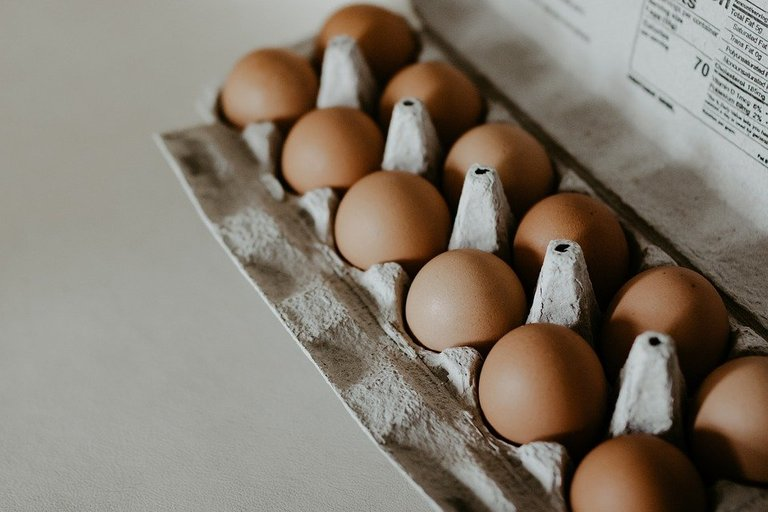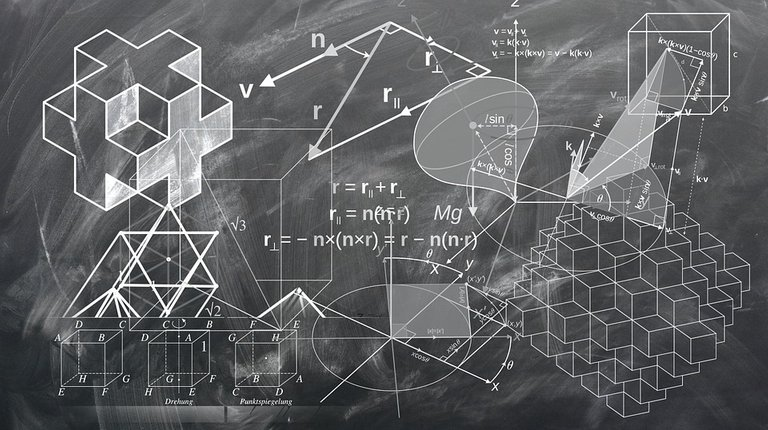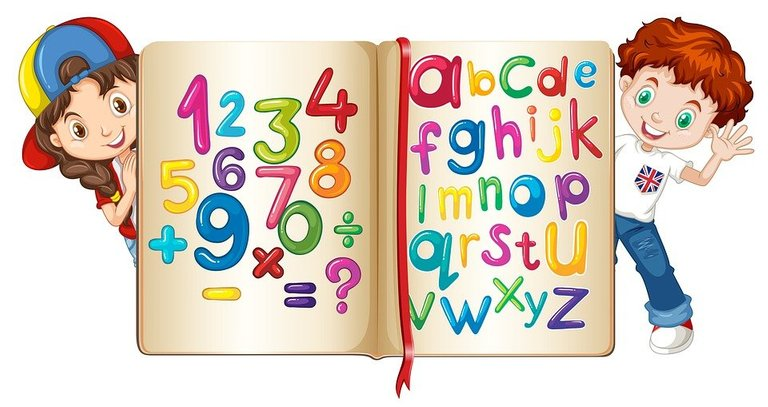# Multiplication Is Important

in Education14 days ago

In this math education post, I want to point out the importance of multiplication. The motivation behind this post is based on a few younger students not liking multiplication in my Mathnasium work. I point out that multiplication shows up in a lot of later mathematics topics and it does show up in some (basic) real world life scenarios.Pixabay Image Source

## Topics

• Multiplication Represented In Groups
• A Prerequisite For Division
• Multiplication Shows Up In Later Math Concepts
• Applications Of Multiplications
• Learning The Times Tables Is Not Fun But It Is Needed

## Multiplication Represented In Groups

Multiplication is based on repeated addition and groups. For example 2 x 5 is based on 5 + 5. There are two fives. Another example of repeated addition would be 3 + 3 + 3 + 3 which would be 4 x 3 as there are 4 threes.

One common grouping of numbers is a dozen. A dozen consists of 12 items. Eggs are often solid in a dozen and the same with donuts. Multiplication is also useful with measurement conversions. For example, one metre is 100 centimetres so five metres would be 5 x 100 or 500 centimetres.Pixabay Image Source

## A Prerequisite For Division

Those who struggle with multiplication will very likely struggle with (long) division. In a way division is the reverse of multiplication. Division can be expressed in a multiplication format. Asking 16 divided by 2 can be rephrased into 2 times something gives 16. Another example would be what is 64 divided by 8? This can be rephrased as 8 times something gives 64.Pixabay Image Source

## Multiplication Shows Up In Later Math Concepts

Looking past division, multiplication is one of the key operations when it comes to algebra. Poor multiplication skills will not help with order of operations, high school algebra, solving linear equations and solving quadratic equations.

One of the unfortunate truths and realities with mathematics and other technical subjects is that is you fall behind you stay behind.Pixabay Image Source

## Applications Of Multiplication

I have briefly mentioned the applications of multiplication with dozens and measurement conversions. There are more applications of multiplication that can be used in real life.

Counting Money

Counting money is one small topic within the large area of personal finance. Being to count money is important for knowing for much money you have, making sure you pay enough money at the grocery store, making sure you receive enough money from the buyer and more.

To calculate something like 10 nickels, note that one nickel is 5 cents. This means that you would do 10 x 5 to obtain 50 cents. An example with higher amounts of money would be 15 \$20 dollar bills. The amount of money in 15 \$20 dollar bills would be 15 x 20 = \$300.

A lot of food packages and boxes contain smaller packs of snacks. Granola bar boxes are often in packs of 5. Four packs of granola bar boxes would contain 20 granola bars (4 x 5).

Packaged juice boxes come in multiples in each package. It is common to see packs of 6 in each juice box package at the grocery store/supermarket. Purchasing five juice box packages yields 30 juice boxes. This is from 5 x 6 = 30.

Multiplication helps a lot in determining costs for flooring materials. Being able to know costs helps decide whether something is afforadable or not.

There is a laminate flooring option that is \$2 CAD per square foot. If a space has 500 square feet. These laminate floor tiles would cost \$1000 from 2 x 500. Keep in mind there are other additional costs such as an underlayment and paying someone to do the flooring.

Those who want nicer flooring may look into hardwood flooring. It is common to see \$5 CAD per square foot. Using this same 500 square feet area the cost of the flooring would be \$2500 from 5 x 500. This is \$1500 more than the laminate flooring option.Pixabay Image Source

## Learning The Times Tables Is Not Fun But It Is Needed

I want to conclude this post by saying that although learning multiplication may not be the most exciting thing ever, it is very important. Sometimes the boring stuff is the most important. Mathematics gets a bad reputation for boring, hard and repetitive tasks such as multiplication. The one thing students should learn and respect is that learning does require a bit of work. As much as there are efforts to try to make learning fun, sometimes we have to struggle a little bit until we get good.Pixabay Image Source